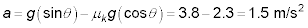##### Physics I Workbook For Dummies with Online Practice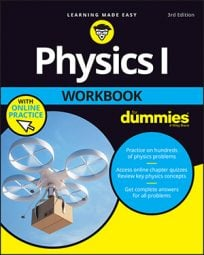Suppose that you have a crate that has been mistakenly placed near the top of a long ramp, and it starts sliding down that ramp. How about calculating its acceleration down the incline?

The object is sliding down the ramp — you’re not pushing it — which means the force of kinetic friction is opposing (not adding to) the component of gravity along the ramp. So the net force on the crate is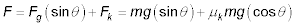Because a = F/m, the acceleration of the crate is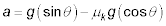Notice that the crate’s mass does not affect its acceleration because mass cancels out of the final equation.

## Sample question

1. A plastic crate slips down a 19-degree ramp with a coefficient of kinetic friction of 0.10. What is its acceleration as it slides?

The correct answer is 2.3 m/s2.

1. You can use the equation2. Plug in the numbers: a = 3.2 – 0.93 = 2.3 m/s2.

## Practice questions

1. You’re dragging a suitcase up a ramp into a luxury hotel when it gets away from you. If the angle of the ramp is 31 degrees and the coefficient of kinetic friction is 0.1, what is the suitcase’s acceleration down the ramp?

2. You drop a 5.0-kg box on a ramp of 12 degrees, and the coefficient of kinetic friction is 0.15. Will the box slide down the ramp?

3. You drop a 1.0-kg book on a 15-degree ramp, and the coefficient of kinetic friction is 0.30. Will the book slide down the ramp?

4. A refrigerator breaks away from the movers and slides down a 23-degree ramp that has a coefficient of kinetic friction of 0.25. What is its acceleration?

Following are answers to the practice questions:

1. 4 m/s2

1. Calculate the forces on the suitcase: The force due to gravity is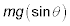and the force due to kinetic friction is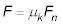You need to find the normal force.

2. The equation for the normal force is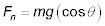Use the normal force to calculate the force due to kinetic friction: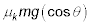3. The net force on the suitcase along the ramp is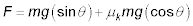4. Divide the net force along the ramp by m to get the acceleration:5. Plug in the numbers: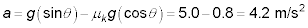2. Yes, the box will slide.

1. Calculate the forces on the box, and if the force down the ramp is larger than the force up the ramp, the box will slide. The force down the ramp is2. The force due to kinetic friction pointing up the ramp iswhich means you need to find the normal force.

3. The equation for the normal force isUse the normal force to calculate the force due to kinetic friction:4. The net force on the suitcase down the ramp is5. Plug in the numbers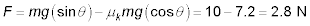down the ramp, so the box will slide.

3. No, the book will not slide.

1. Calculate the forces on the book, and if the force down the ramp is larger than the force up the ramp, the book will slide. The force down the ramp is2. The force due to kinetic friction pointing up the ramp iswhich means you need to find the normal force.

3. The equation for the normal force isUse the normal force to calculate the force due to kinetic friction:4. The net force on the book down the ramp is5. Plug in the numbers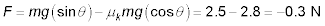along the ramp, so the book will not slide.

4. 1.5 m/s2 down the ramp

1. Calculate the forces on the refrigerator: The force down the ramp isand the force due to kinetic friction pointing up the ramp isYou need to find the normal force.

2. The equation for the normal force isUse the normal force to calculate the force due to kinetic friction:3. The net force on the refrigerator down the ramp is4. Divide by m to get the acceleration:5. Plug in the numbers: